# RD Sharma Solutions for Class 10 Maths Chapter 16 Surface Areas And Volumes Exercise 16.1

Just like the previous chapter, this one deals with surface areas and volumes of solids. This exercise consists of problems on the conversion of solids from one type to the other. Students can make use of the RD Sharma Solutions Class 10 to clarify any doubts regarding this chapter. Further, the RD Sharma Solutions for Class 10 Maths Chapter 16 Surface Areas And Volumes Exercise 16.1 PDF can be downloaded below.

## RD Sharma Solutions for Class 10 Maths Chapter 16 Surface Areas And Volumes Exercise 16.1 Download PDF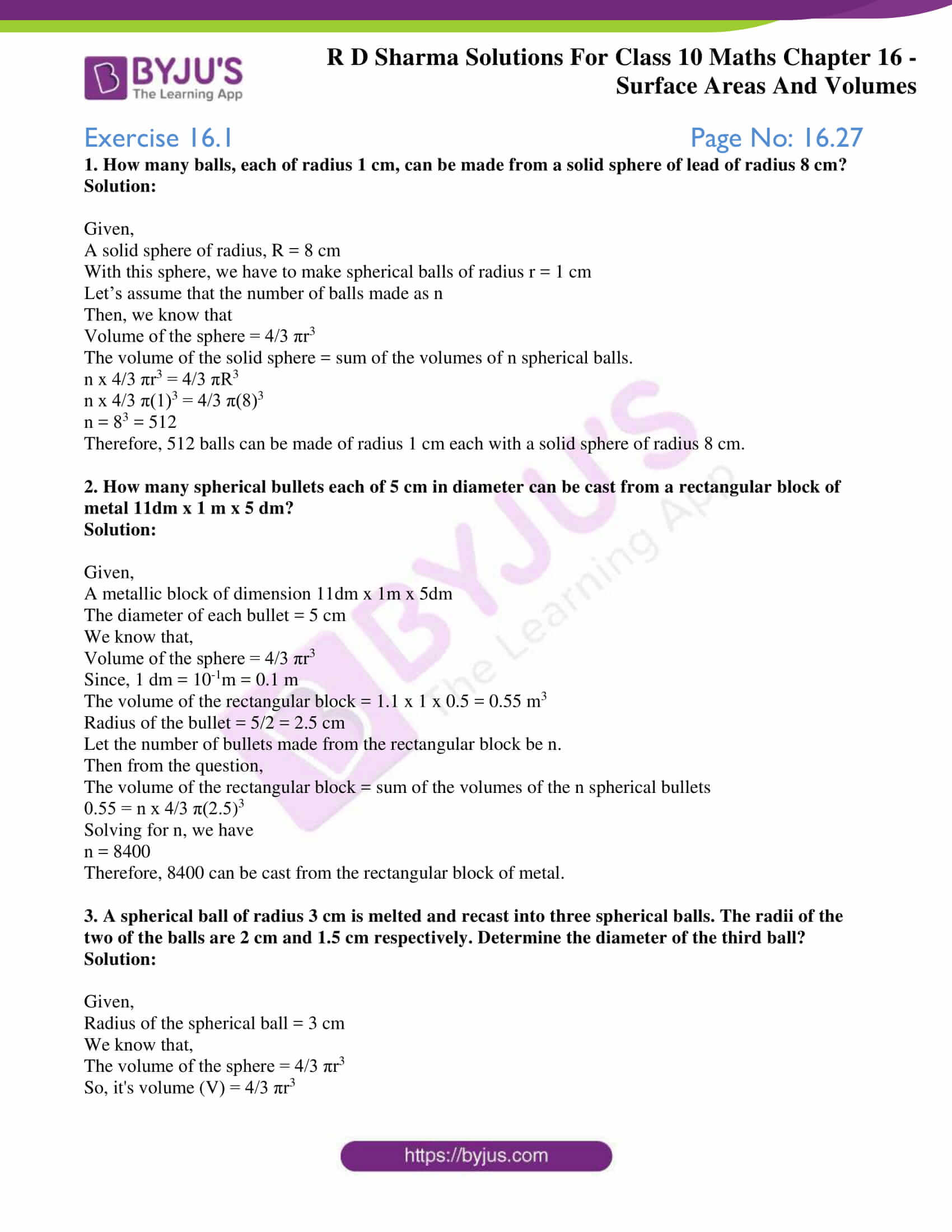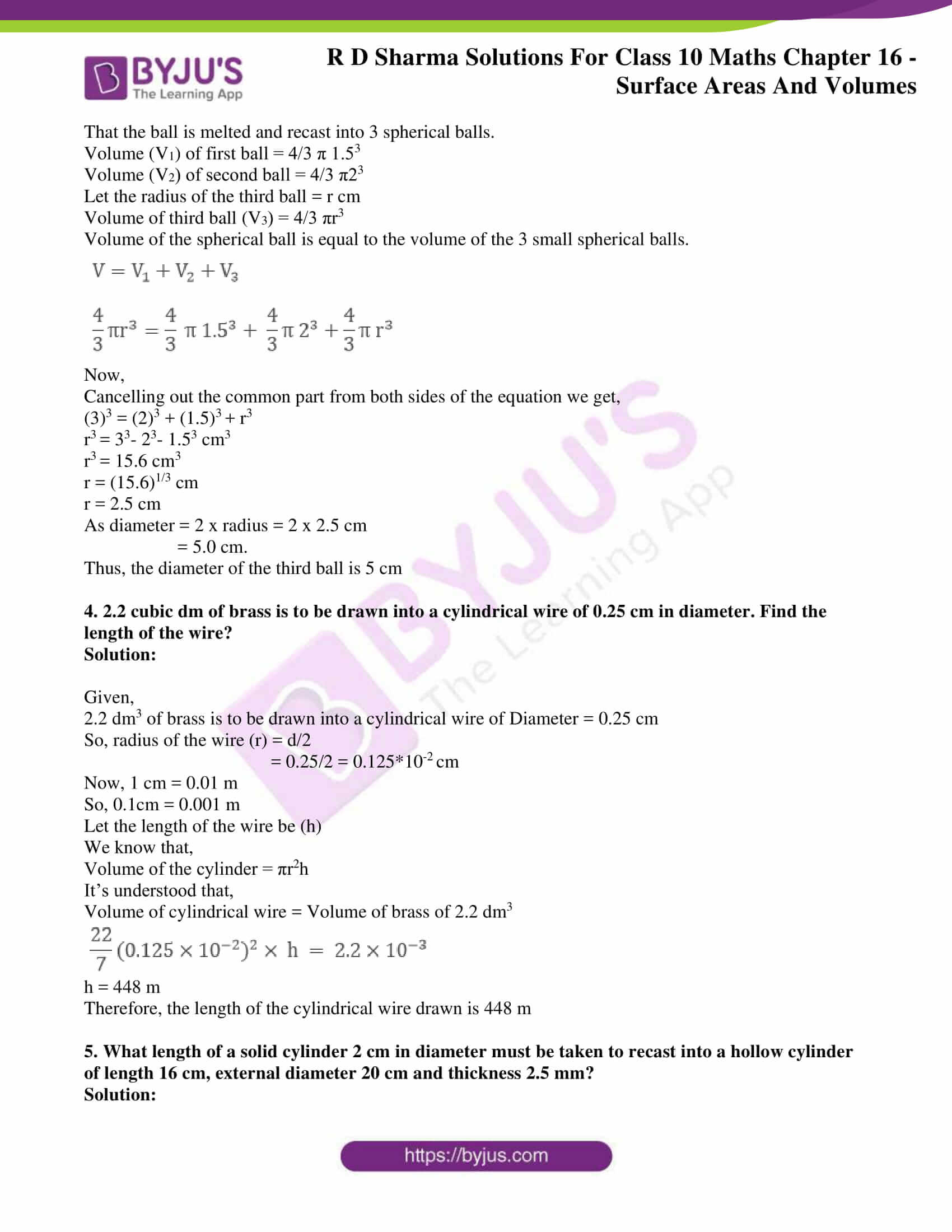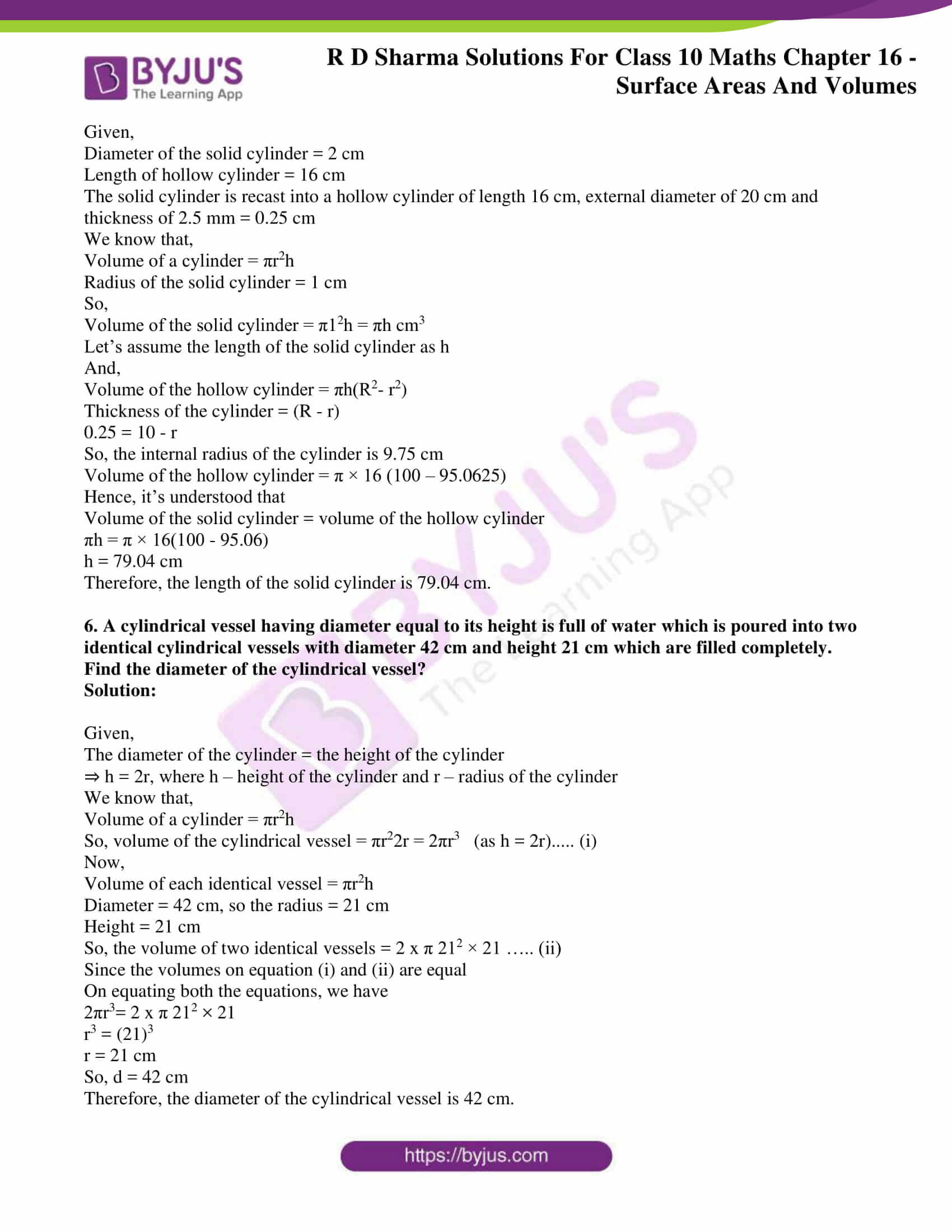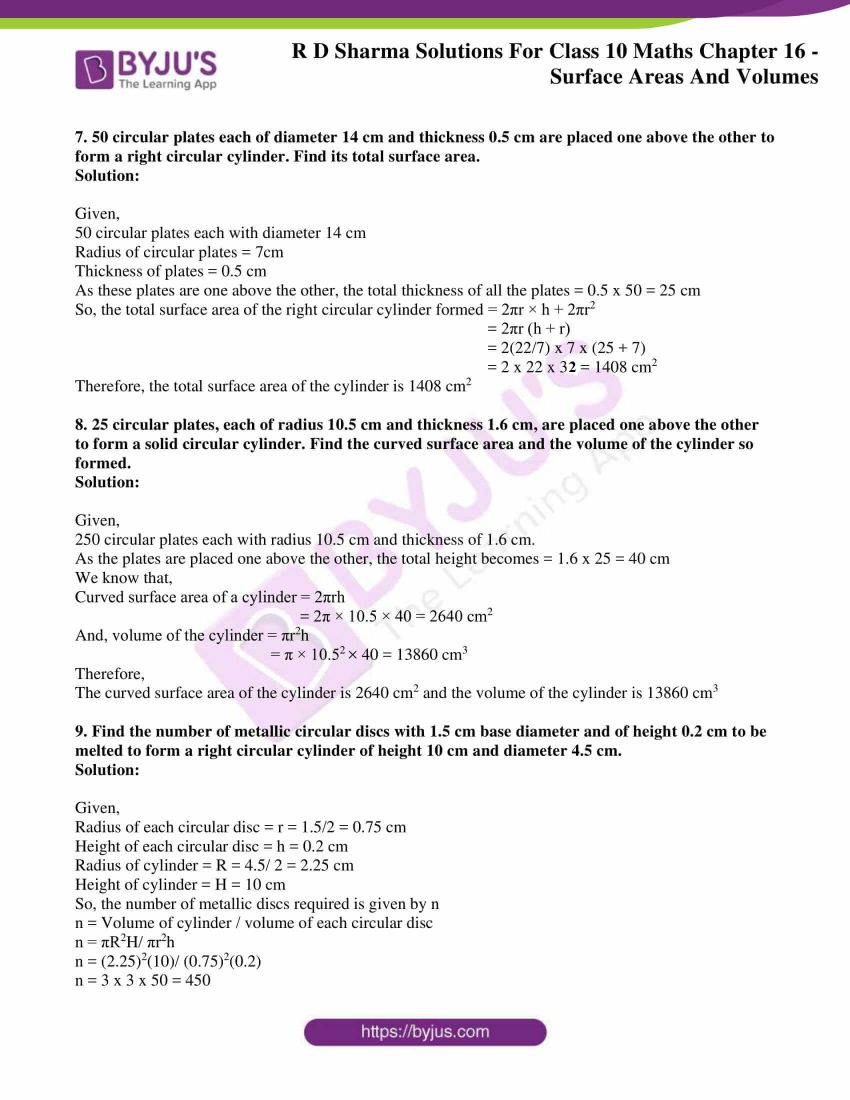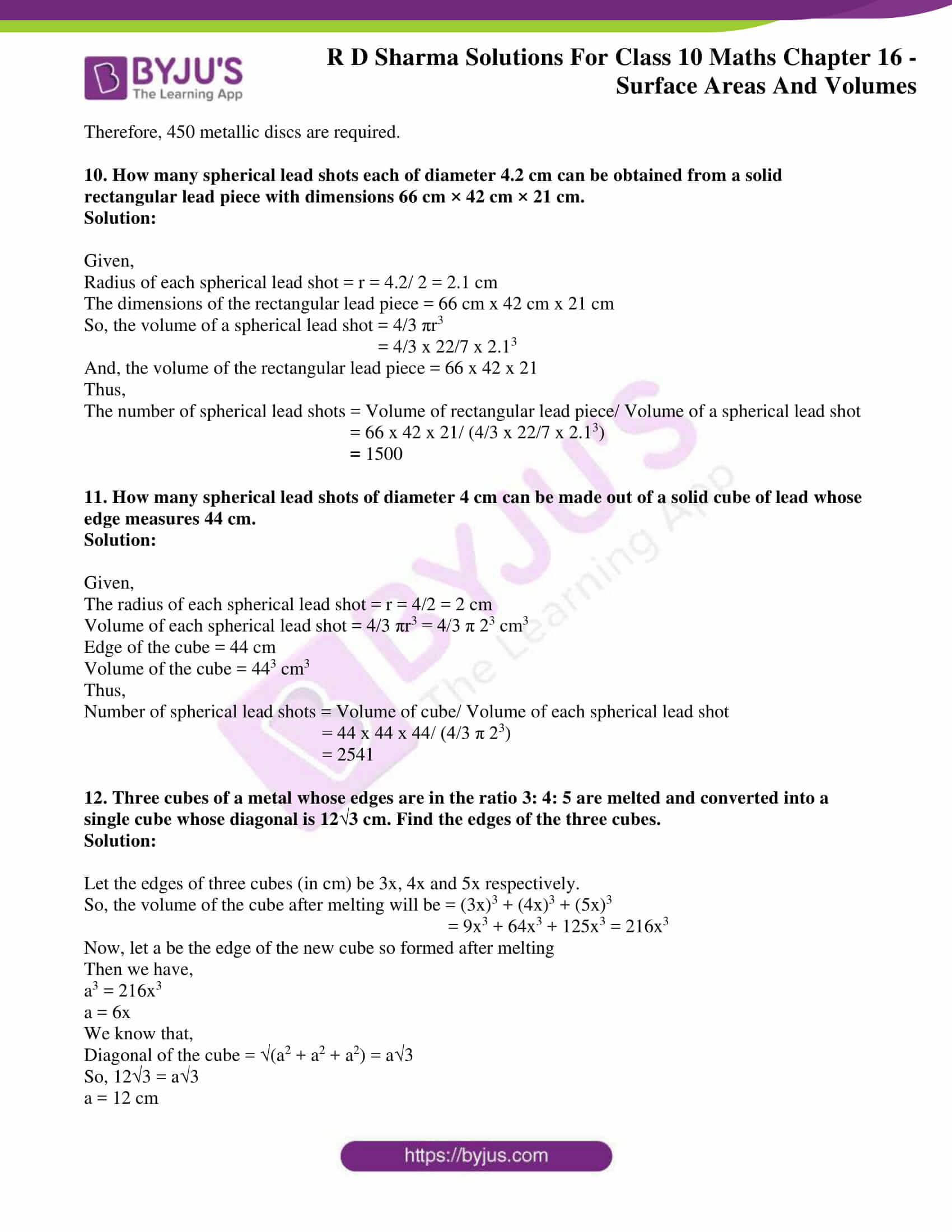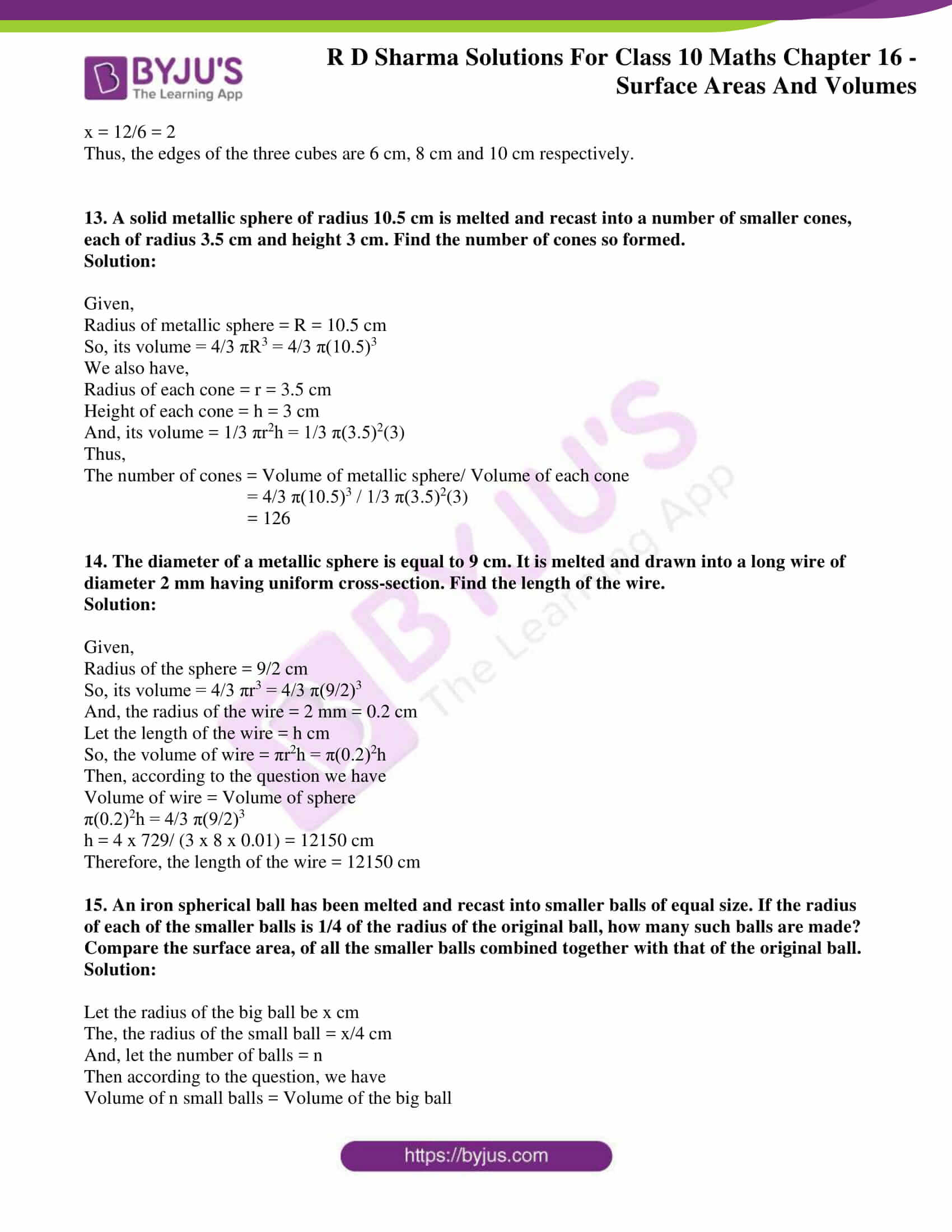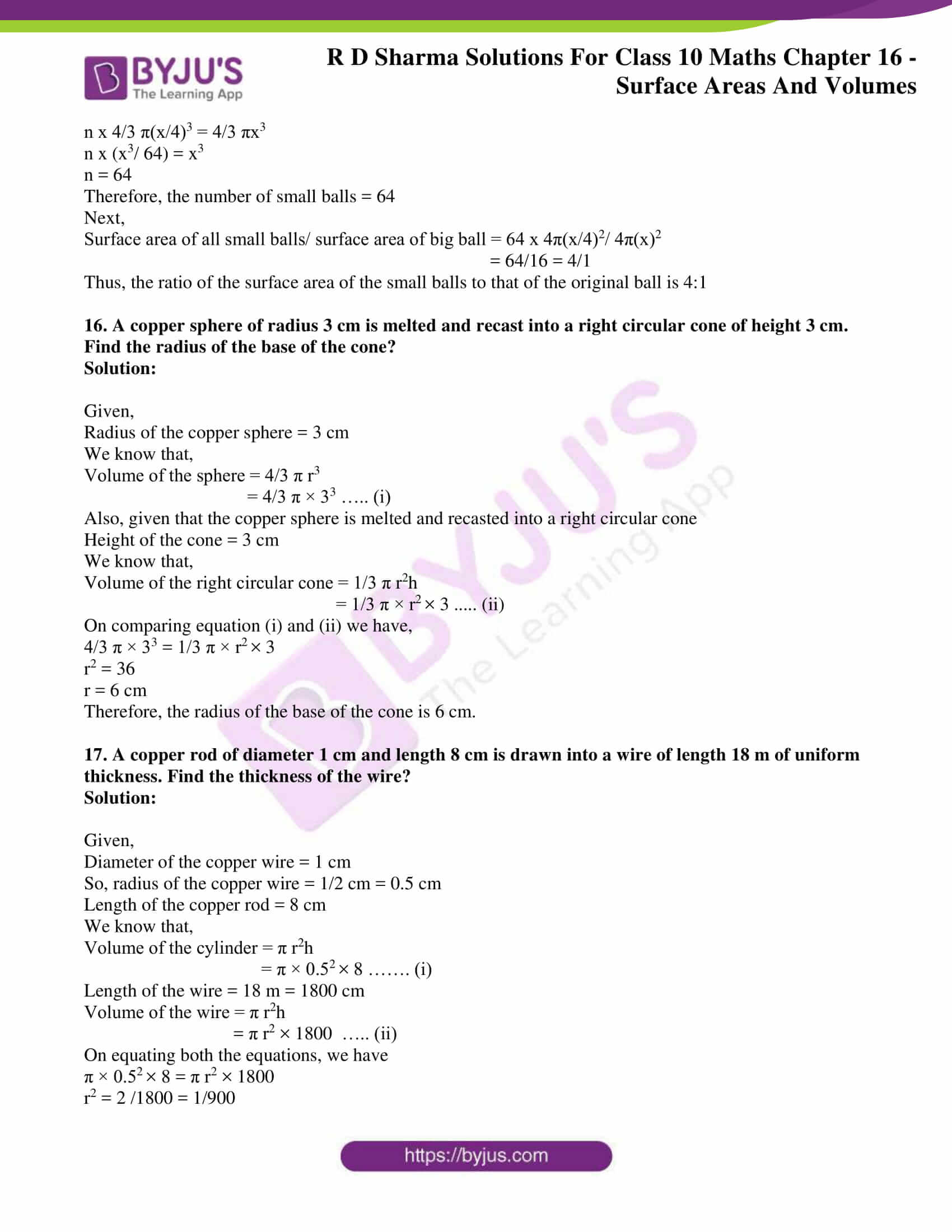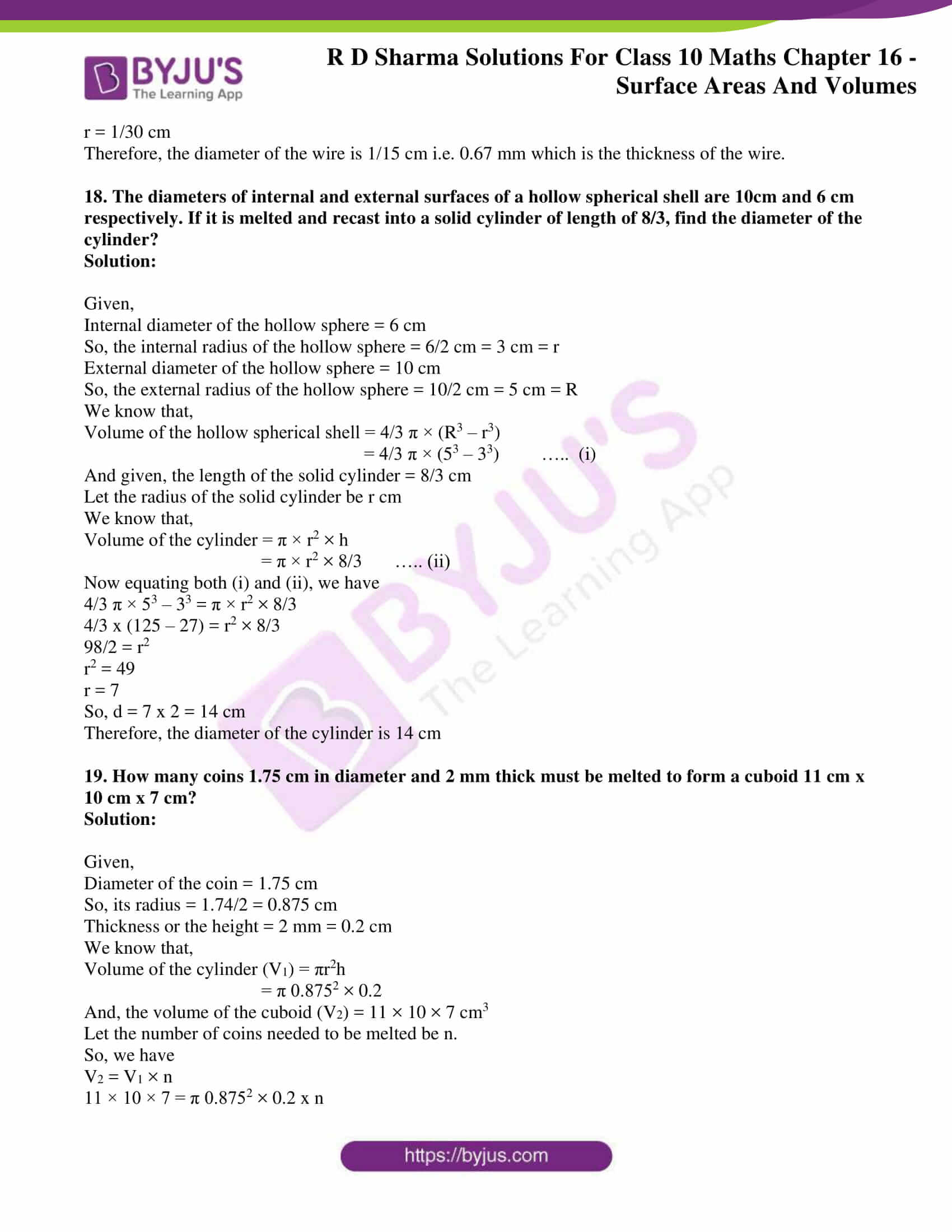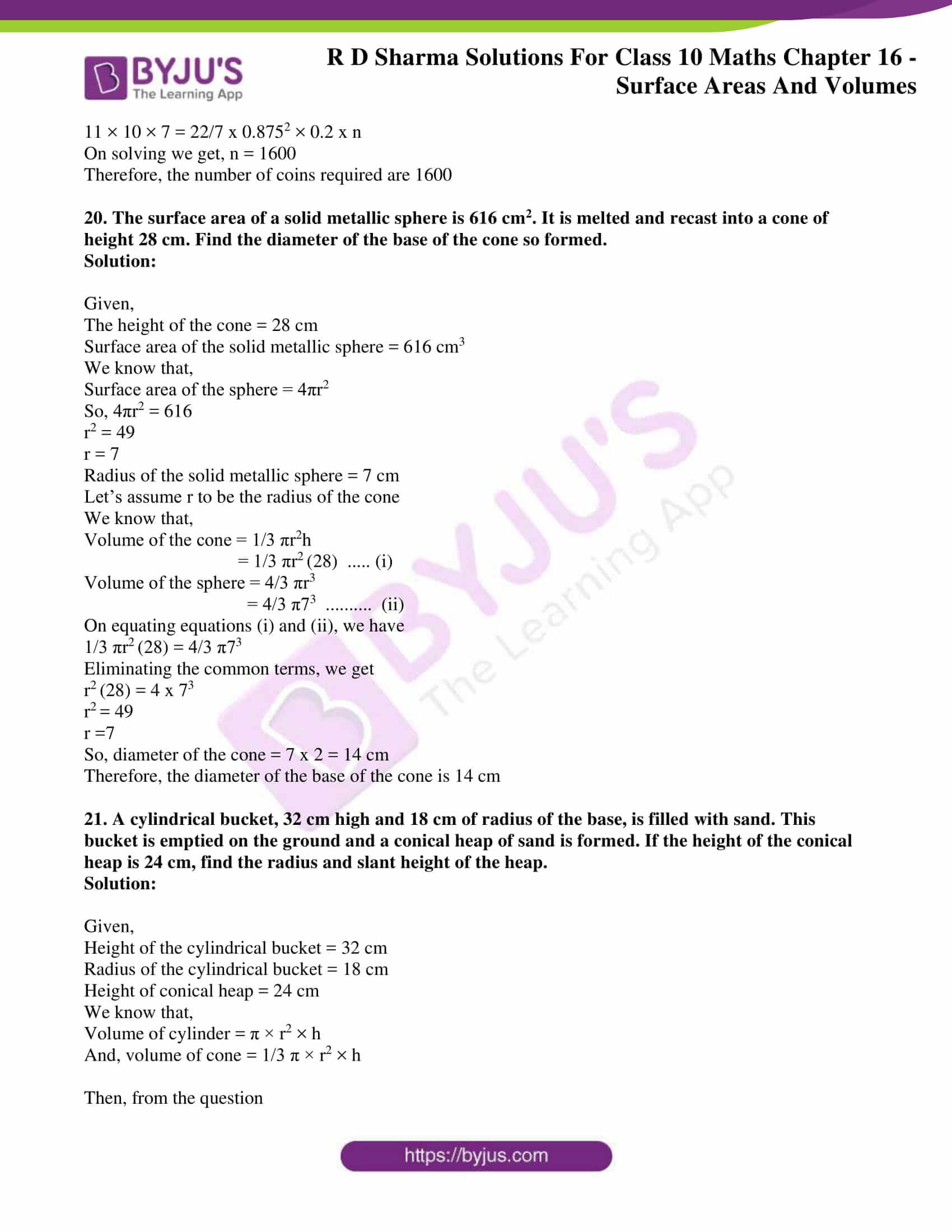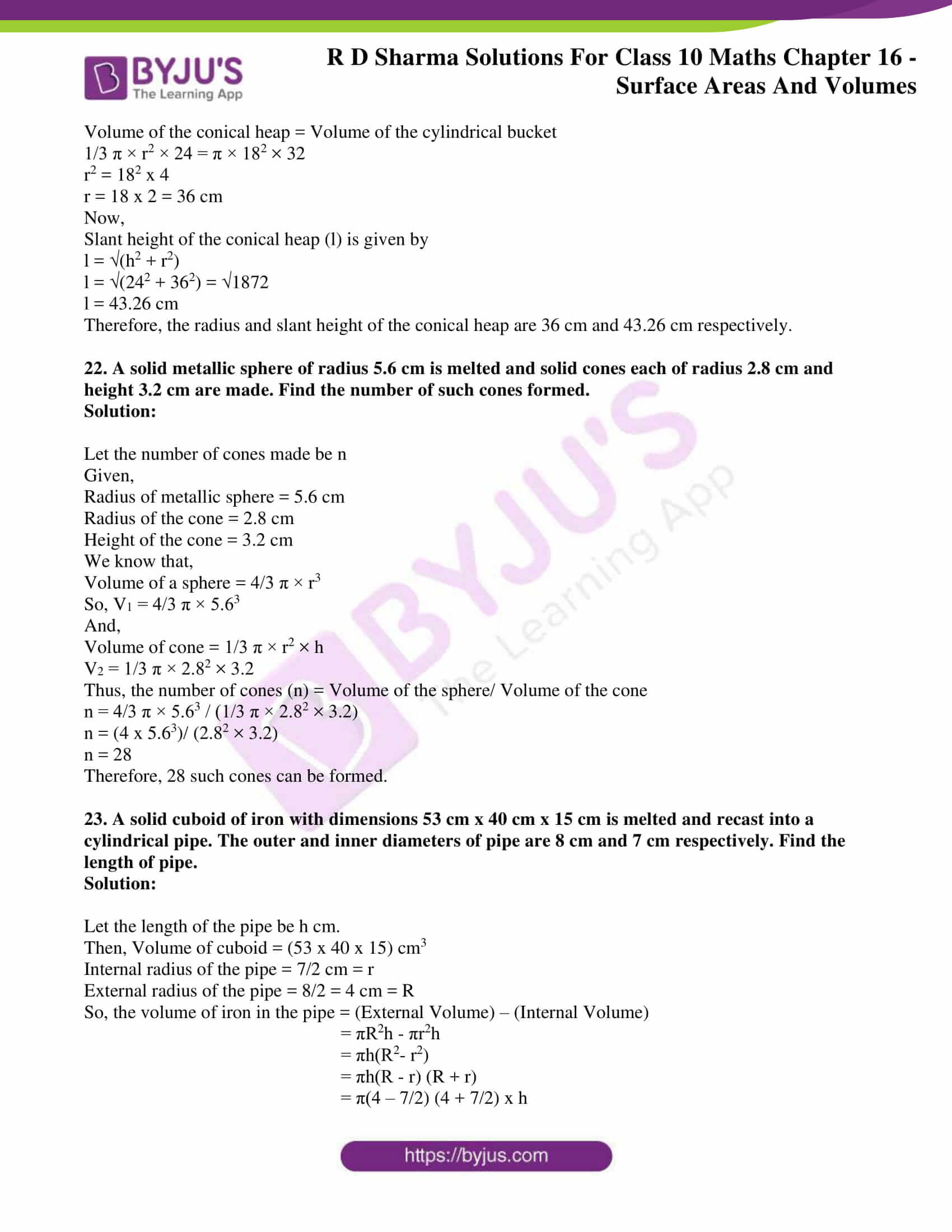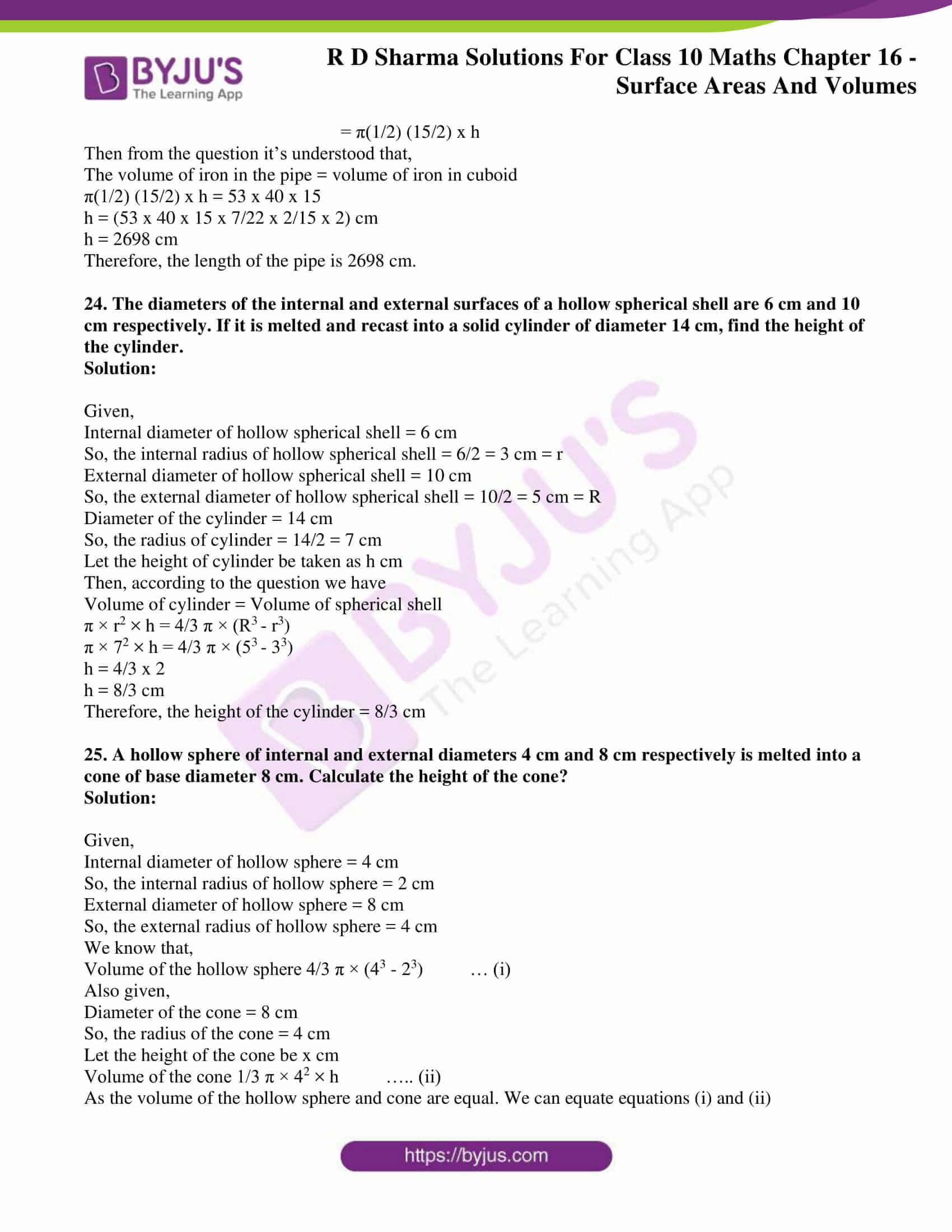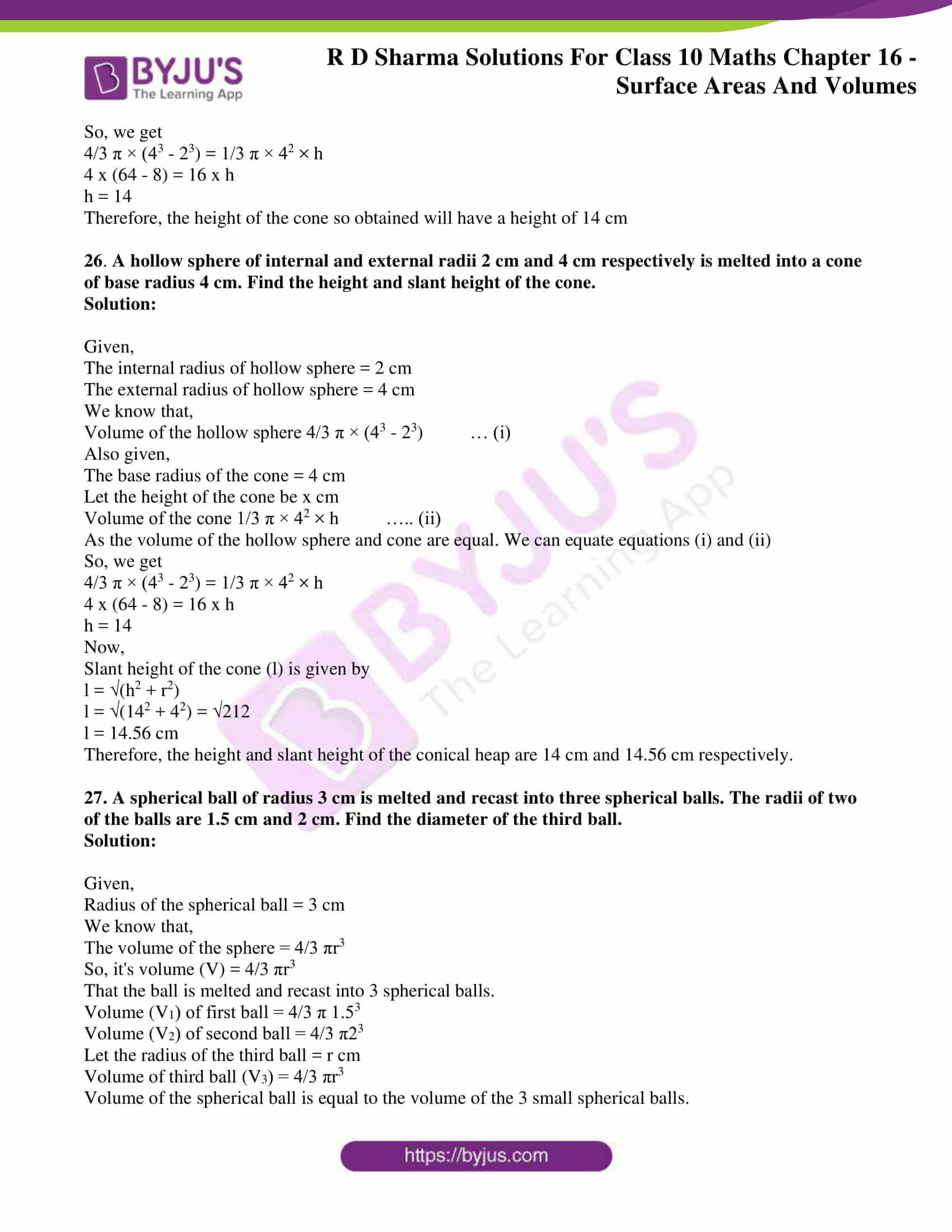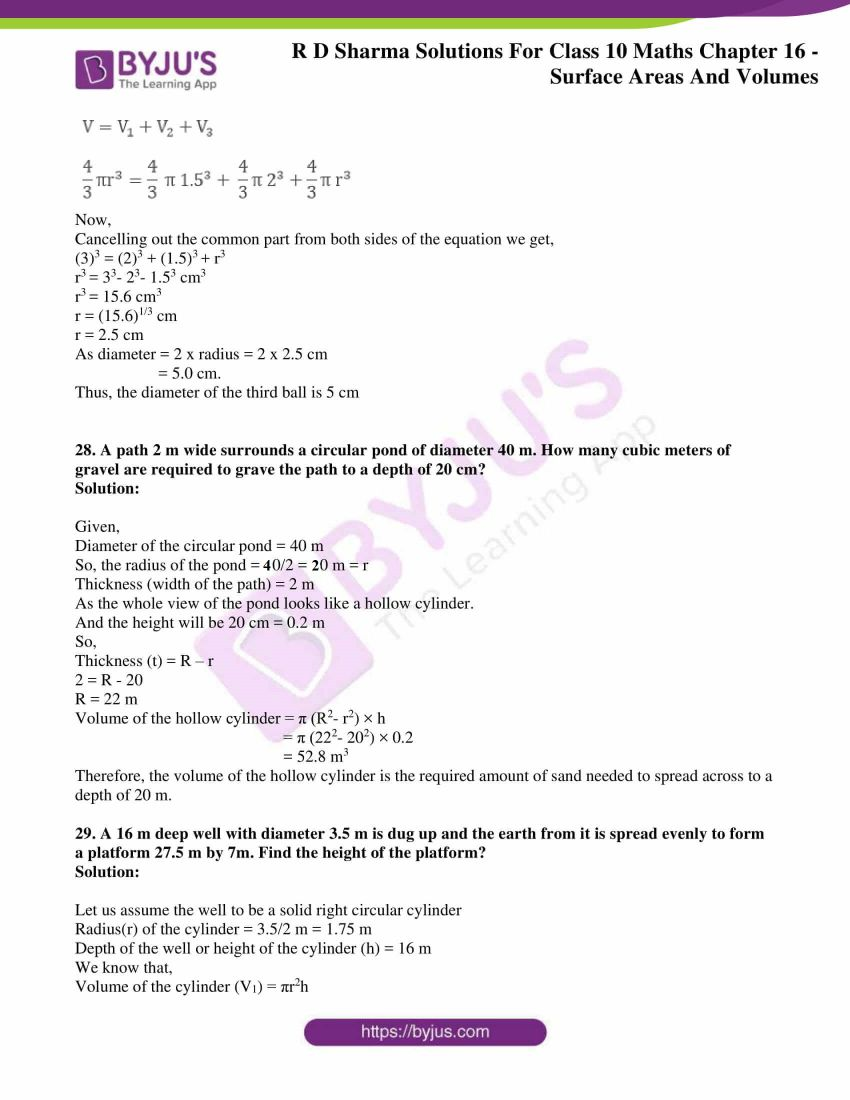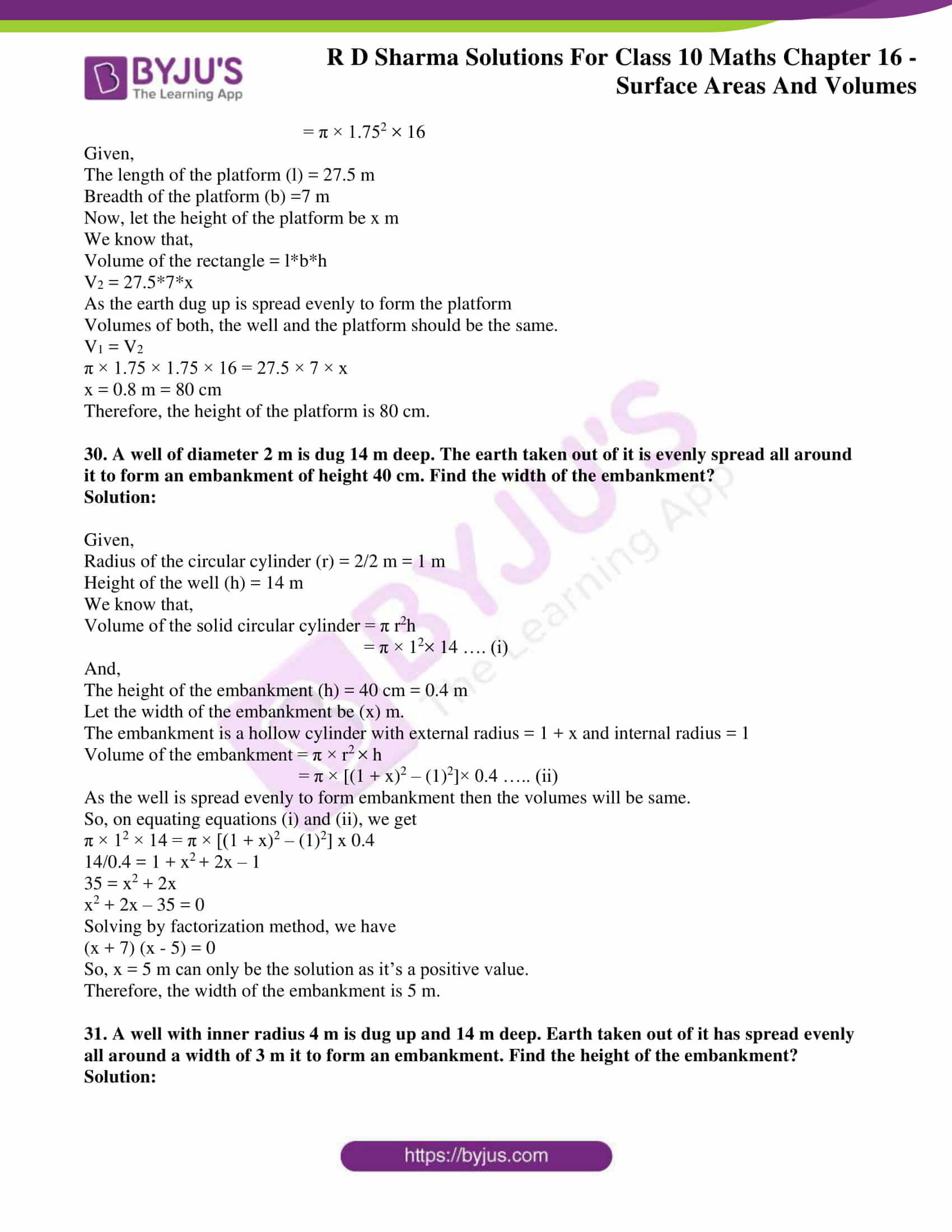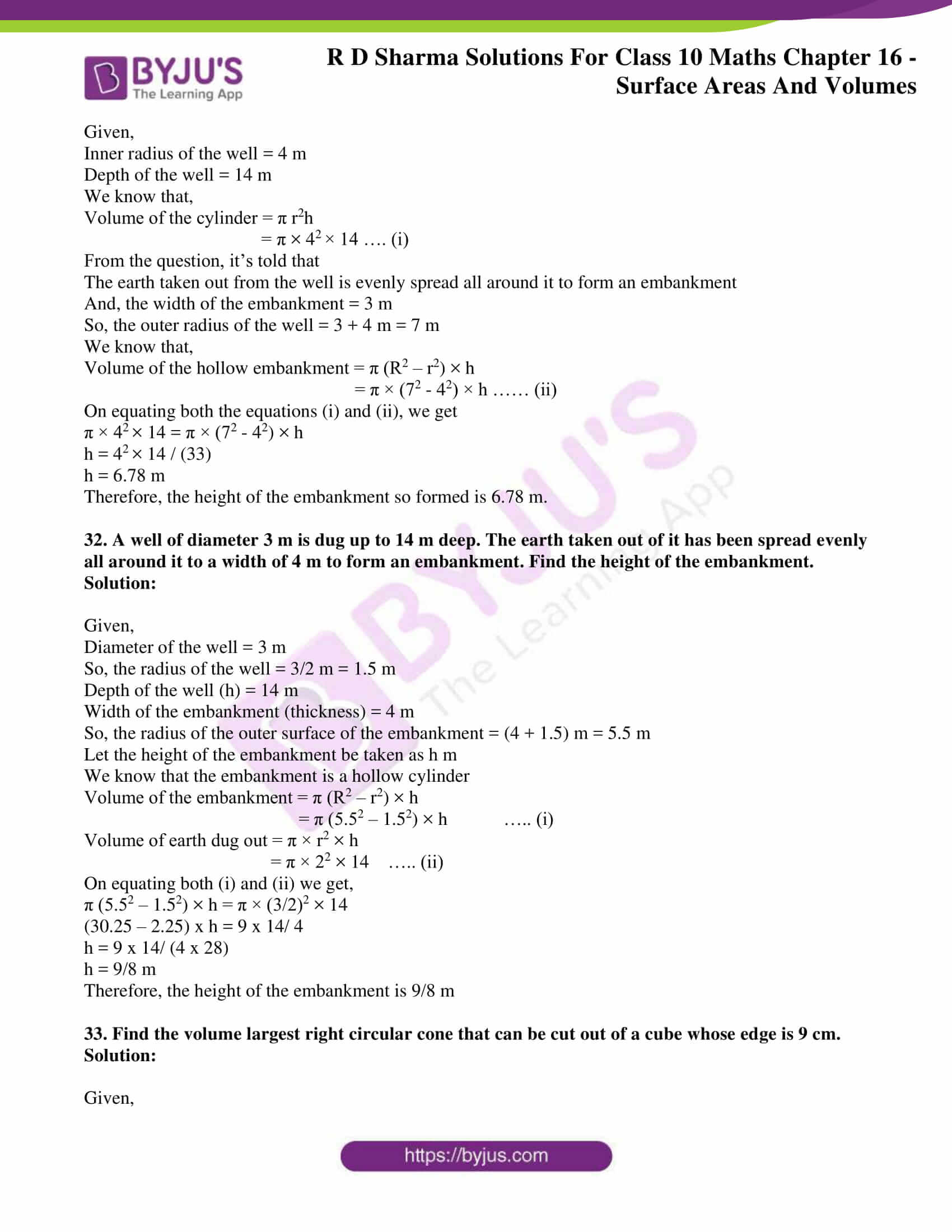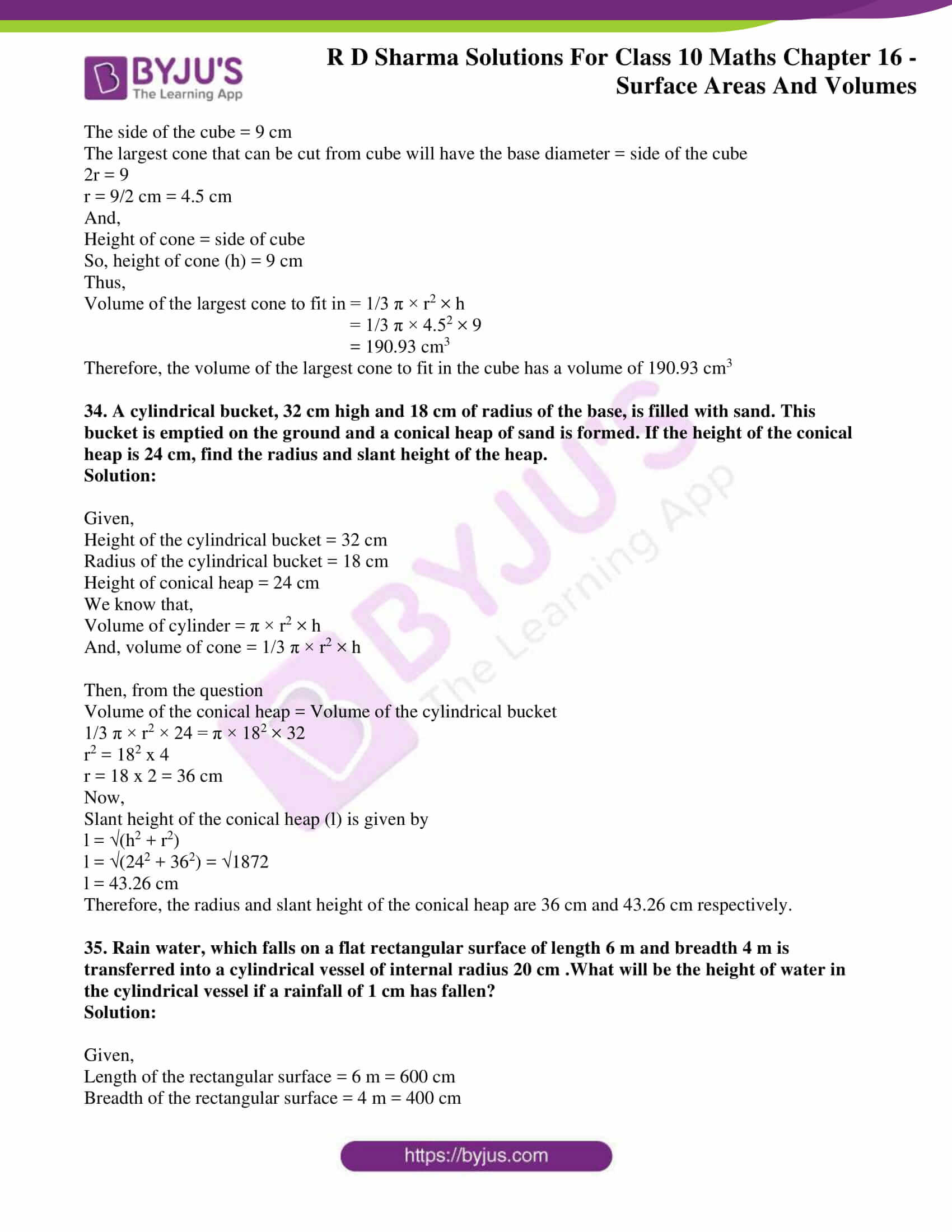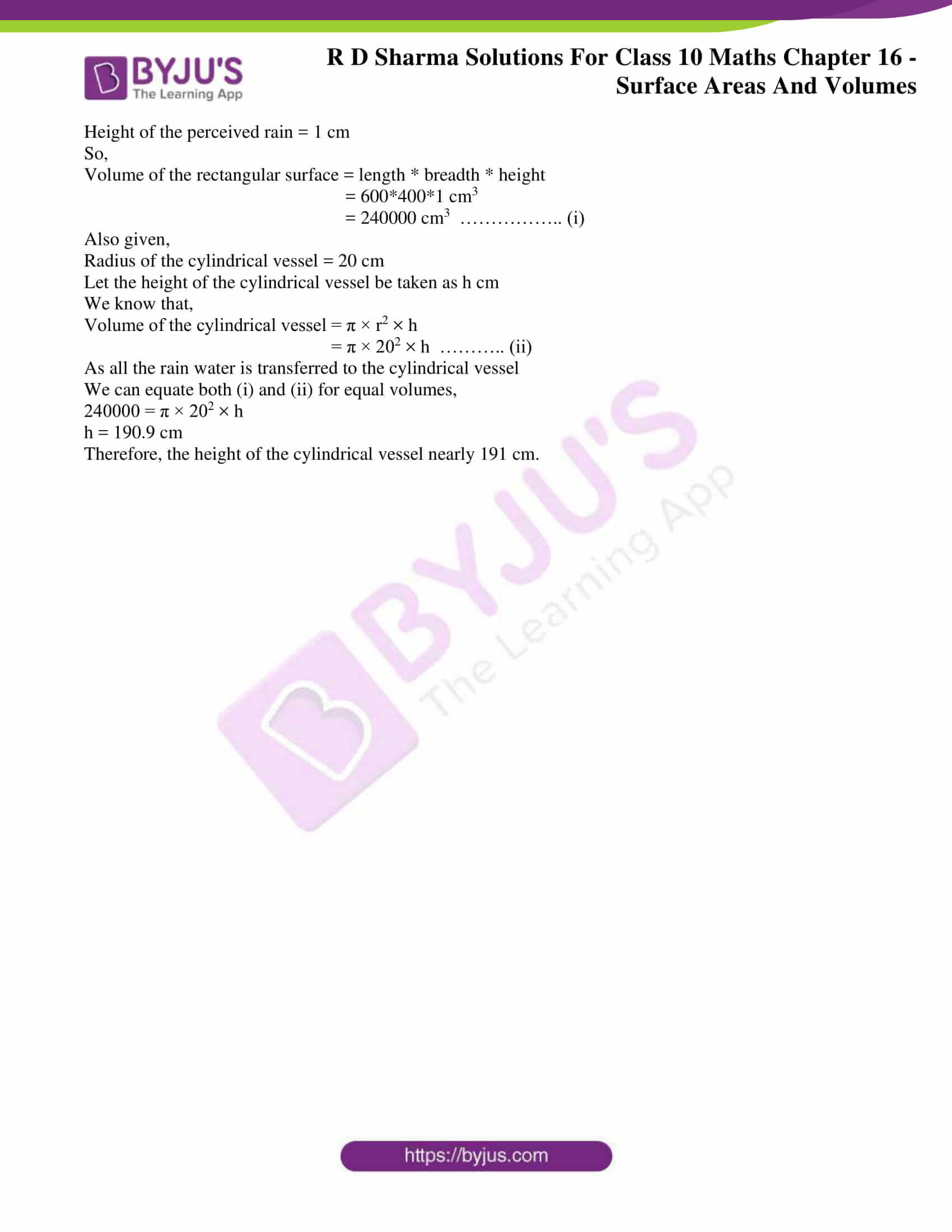### Access RD Sharma Solutions for Class 10 Maths Chapter 16 Surface Areas And Volumes Exercise 16.1

1. How many balls, each of radius 1 cm, can be made from a solid sphere of lead of radius 8 cm?

Solution:

Given,

A solid sphere of radius, R = 8 cm

With this sphere, we have to make spherical balls of radius r = 1 cm

Let’s assume that the number of balls made as n

Then, we know that

Volume of the sphere = 4/3 πr3

The volume of the solid sphere = sum of the volumes of n spherical balls.

n x 4/3 πr3 = 4/3 πR3

n x 4/3 π(1)3 = 4/3 π(8)3

n = 83 = 512

Therefore, 512 balls can be made of radius 1 cm each with a solid sphere of radius 8 cm.

2. How many spherical bullets each of 5 cm in diameter can be cast from a rectangular block of metal 11dm x 1 m x 5 dm?

Solution:

Given,

A metallic block of dimension 11dm x 1m x 5dm

The diameter of each bullet = 5 cm

We know that,

Volume of the sphere = 4/3 πr3

Since, 1 dm = 10-1m = 0.1 m

The volume of the rectangular block = 1.1 x 1 x 0.5 = 0.55 m3

Radius of the bullet = 5/2 = 2.5 cm

Let the number of bullets made from the rectangular block be n.

Then from the question,

The volume of the rectangular block = sum of the volumes of the n spherical bullets

0.55 = n x 4/3 π(2.5)3

Solving for n, we have

n = 8400

Therefore, 8400 can be cast from the rectangular block of metal.

3. A spherical ball of radius 3 cm is melted and recast into three spherical balls. The radii of the two of the balls are 2 cm and 1.5 cm respectively. Determine the diameter of the third ball?

Solution:

Given,

Radius of the spherical ball = 3 cm

We know that,

The volume of the sphere = 4/3 πr3

So, it’s volume (V) = 4/3 πr3

That the ball is melted and recast into 3 spherical balls.

Volume (V1) of first ball = 4/3 π 1.53

Volume (V2) of second ball = 4/3 π23

Let the radius of the third ball = r cm

Volume of third ball (V3) = 4/3 πr3

Volume of the spherical ball is equal to the volume of the 3 small spherical balls.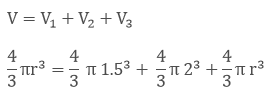Now,

Cancelling out the common part from both sides of the equation we get,

(3)3 = (2)3 + (1.5)+ r3

r= 33– 23– 1.53 cm3

r= 15.6 cm3

r = (15.6)1/3 cm

r = 2.5 cm

As diameter = 2 x radius = 2 x 2.5 cm

= 5.0 cm.

Thus, the diameter of the third ball is 5 cm

4. 2.2 cubic dm of brass is to be drawn into a cylindrical wire of 0.25 cm in diameter. Find the length of the wire?

Solution:

Given,

2.2 dm3 of brass is to be drawn into a cylindrical wire of Diameter = 0.25 cm

So, radius of the wire (r) = d/2

= 0.25/2 = 0.125*10-2 cm

Now, 1 cm = 0.01 m

So, 0.1cm = 0.001 m

Let the length of the wire be (h)

We know that,

Volume of the cylinder = πr2h

It’s understood that,

Volume of cylindrical wire = Volume of brass of 2.2 dm3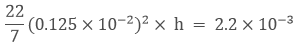h = 448 m

Therefore, the length of the cylindrical wire drawn is 448 m

5. What length of a solid cylinder 2 cm in diameter must be taken to recast into a hollow cylinder of length 16 cm, external diameter 20 cm and thickness 2.5 mm?

Solution:

Given,

Diameter of the solid cylinder = 2 cm

Length of hollow cylinder = 16 cm

The solid cylinder is recast into a hollow cylinder of length 16 cm, external diameter of 20 cm and thickness of 2.5 mm = 0.25 cm

We know that,

Volume of a cylinder = πr2h

Radius of the solid cylinder = 1 cm

So,

Volume of the solid cylinder = π12h = πh cm3

Let’s assume the length of the solid cylinder as h

And,

Volume of the hollow cylinder = πh(R2– r2)

Thickness of the cylinder = (R – r)

0.25 = 10 – r

So, the internal radius of the cylinder is 9.75 cm

Volume of the hollow cylinder = π × 16 (100 – 95.0625)

Hence, it’s understood that

Volume of the solid cylinder = volume of the hollow cylinder

πh = π × 16(100 – 95.06)

h = 79.04 cm

Therefore, the length of the solid cylinder is 79.04 cm.

6. A cylindrical vessel having diameter equal to its height is full of water which is poured into two identical cylindrical vessels with diameter 42 cm and height 21 cm which are filled completely. Find the diameter of the cylindrical vessel?

Solution:

Given,

The diameter of the cylinder = the height of the cylinder

⇒ h = 2r, where h – height of the cylinder and r – radius of the cylinder

We know that,

Volume of a cylinder = πr2h

So, volume of the cylindrical vessel = πr22r = 2πr3   (as h = 2r)….. (i)

Now,

Volume of each identical vessel = πr2h

Diameter = 42 cm, so the radius = 21 cm

Height = 21 cm

So, the volume of two identical vessels = 2 x π 212 × 21 ….. (ii)

Since the volumes on equation (i) and (ii) are equal

On equating both the equations, we have

2πr3= 2 x π 212 × 21

r3 = (21)3

r = 21 cm

So, d = 42 cm

Therefore, the diameter of the cylindrical vessel is 42 cm.

7. 50 circular plates each of diameter 14 cm and thickness 0.5 cm are placed one above the other to form a right circular cylinder. Find its total surface area.

Solution:

Given,

50 circular plates each with diameter 14 cm

Radius of circular plates = 7cm

Thickness of plates = 0.5 cm

As these plates are one above the other, the total thickness of all the plates = 0.5 x 50 = 25 cm

So, the total surface area of the right circular cylinder formed = 2πr × h + 2πr2

= 2πr (h + r)

= 2(22/7) x 7 x (25 + 7)

= 2 x 22 x 32 = 1408 cm2

Therefore, the total surface area of the cylinder is 1408 cm2

8. 25 circular plates, each of radius 10.5 cm and thickness 1.6 cm, are placed one above the other to form a solid circular cylinder. Find the curved surface area and the volume of the cylinder so formed.

Solution:

Given,

250 circular plates each with radius 10.5 cm and thickness of 1.6 cm.

As the plates are placed one above the other, the total height becomes = 1.6 x 25 = 40 cm

We know that,

Curved surface area of a cylinder = 2πrh

= 2π × 10.5 × 40 = 2640 cm2

And, volume of the cylinder = πr2h

= π × 10.5× 40 = 13860 cm3

Therefore,

The curved surface area of the cylinder is 2640 cm2 and the volume of the cylinder is 13860 cm3

9. Find the number of metallic circular discs with 1.5 cm base diameter and of height 0.2 cm to be melted to form a right circular cylinder of height 10 cm and diameter 4.5 cm.

Solution:

Given,

Radius of each circular disc = r = 1.5/2 = 0.75 cm

Height of each circular disc = h = 0.2 cm

Radius of cylinder = R = 4.5/ 2 = 2.25 cm

Height of cylinder = H = 10 cm

So, the number of metallic discs required is given by n

n = Volume of cylinder / volume of each circular disc

n = πR2H/ πr2h

n = (2.25)2(10)/ (0.75)2(0.2)

n = 3 x 3 x 50 = 450

Therefore, 450 metallic discs are required.

10. How many spherical lead shots each of diameter 4.2 cm can be obtained from a solid rectangular lead piece with dimensions 66 cm × 42 cm × 21 cm.

Solution:

Given,

Radius of each spherical lead shot = r = 4.2/ 2 = 2.1 cm

The dimensions of the rectangular lead piece = 66 cm x 42 cm x 21 cm

So, the volume of a spherical lead shot = 4/3 πr3

= 4/3 x 22/7 x 2.13

And, the volume of the rectangular lead piece = 66 x 42 x 21

Thus,

The number of spherical lead shots = Volume of rectangular lead piece/ Volume of a spherical lead shot

= 66 x 42 x 21/ (4/3 x 22/7 x 2.13)

= 1500

11. How many spherical lead shots of diameter 4 cm can be made out of a solid cube of lead whose edge measures 44 cm.

Solution:

Given,

The radius of each spherical lead shot = r = 4/2 = 2 cm

Volume of each spherical lead shot = 4/3 πr3 = 4/3 π 23 cm3

Edge of the cube = 44 cm

Volume of the cube = 443 cm3

Thus,

Number of spherical lead shots = Volume of cube/ Volume of each spherical lead shot

= 44 x 44 x 44/ (4/3 π 23)

= 2541

12. Three cubes of a metal whose edges are in the ratio 3: 4: 5 are melted and converted into a single cube whose diagonal is 12√3 cm. Find the edges of the three cubes.

Solution:

Let the edges of three cubes (in cm) be 3x, 4x and 5x respectively.

So, the volume of the cube after melting will be = (3x)3 + (4x)3 + (5x)3

= 9x3 + 64x3 + 125x3 = 216x3

Now, let a be the edge of the new cube so formed after melting

Then we have,

a3 = 216x3

a = 6x

We know that,

Diagonal of the cube = (a2 + a2 + a2) = a3

So, 123 = a3

a = 12 cm

x = 12/6 = 2

Thus, the edges of the three cubes are 6 cm, 8 cm and 10 cm respectively.

13. A solid metallic sphere of radius 10.5 cm is melted and recast into a number of smaller cones, each of radius 3.5 cm and height 3 cm. Find the number of cones so formed.

Solution:

Given,

Radius of metallic sphere = R = 10.5 cm

So, its volume = 4/3 πR3 = 4/3 π(10.5)3

We also have,

Radius of each cone = r = 3.5 cm

Height of each cone = h = 3 cm

And, its volume = 1/3 πr2h = 1/3 π(3.5)2(3)

Thus,

The number of cones = Volume of metallic sphere/ Volume of each cone

= 4/3 π(10.5)3 / 1/3 π(3.5)2(3)

= 126

14. The diameter of a metallic sphere is equal to 9 cm. It is melted and drawn into a long wire of diameter 2 mm having uniform cross-section. Find the length of the wire.

Solution:

Given,

Radius of the sphere = 9/2 cm

So, its volume = 4/3 πr3 = 4/3 π(9/2)3

And, the radius of the wire = 2 mm = 0.2 cm

Let the length of the wire = h cm

So, the volume of wire = πr2h = π(0.2)2h

Then, according to the question we have

Volume of wire = Volume of sphere

π(0.2)2h = 4/3 π(9/2)3

h = 4 x 729/ (3 x 8 x 0.01) = 12150 cm

Therefore, the length of the wire = 12150 cm

15. An iron spherical ball has been melted and recast into smaller balls of equal size. If the radius of each of the smaller balls is 1/4 of the radius of the original ball, how many such balls are made? Compare the surface area, of all the smaller balls combined together with that of the original ball.

Solution:

Let the radius of the big ball be x cm

The, the radius of the small ball = x/4 cm

And, let the number of balls = n

Then according to the question, we have

Volume of n small balls = Volume of the big ball

n x 4/3 π(x/4)3 = 4/3 πx3

n x (x3/ 64) = x3

n = 64

Therefore, the number of small balls = 64

Next,

Surface area of all small balls/ surface area of big ball = 64 x 4π(x/4)2/ 4π(x)2

= 64/16 = 4/1

Thus, the ratio of the surface area of the small balls to that of the original ball is 4:1

16. A copper sphere of radius 3 cm is melted and recast into a right circular cone of height 3 cm. Find the radius of the base of the cone?

Solution:

Given,

Radius of the copper sphere = 3 cm

We know that,

Volume of the sphere = 4/3 π r3

= 4/3 π × 33 ….. (i)

Also, given that the copper sphere is melted and recasted into a right circular cone

Height of the cone = 3 cm

We know that,

Volume of the right circular cone = 1/3 π r2h

= 1/3 π × r× 3 ….. (ii)

On comparing equation (i) and (ii) we have,

4/3 π × 33 = 1/3 π × r× 3

r2 = 36

r = 6 cm

Therefore, the radius of the base of the cone is 6 cm.

17. A copper rod of diameter 1 cm and length 8 cm is drawn into a wire of length 18 m of uniform thickness. Find the thickness of the wire?

Solution:

Given,

Diameter of the copper wire = 1 cm

So, radius of the copper wire = 1/2 cm = 0.5 cm

Length of the copper rod = 8 cm

We know that,

Volume of the cylinder = π r2h

= π × 0.5× 8 ……. (i)

Length of the wire = 18 m = 1800 cm

Volume of the wire = π r2h

= π r2 × 1800  ….. (ii)

On equating both the equations, we have

π × 0.5× 8 = π r2 × 1800

r2 = 2 /1800 = 1/900

r = 1/30 cm

Therefore, the diameter of the wire is 1/15 cm i.e. 0.67 mm which is the thickness of the wire.

18. The diameters of internal and external surfaces of a hollow spherical shell are 10cm and 6 cm respectively. If it is melted and recast into a solid cylinder of length of 8/3, find the diameter of the cylinder?

Solution:

Given,

Internal diameter of the hollow sphere = 6 cm

So, the internal radius of the hollow sphere = 6/2 cm = 3 cm = r

External diameter of the hollow sphere = 10 cm

So, the external radius of the hollow sphere = 10/2 cm = 5 cm = R

We know that,

Volume of the hollow spherical shell = 4/3 π × (R3 – r3)

= 4/3 π × (53 – 33)         …..  (i)

And given, the length of the solid cylinder = 8/3 cm

Let the radius of the solid cylinder be r cm

We know that,

Volume of the cylinder = π × r2 × h

= π × r2 × 8/3       ….. (ii)

Now equating both (i) and (ii), we have

4/3 π × 53 – 33 = π × r2 × 8/3

4/3 x (125 – 27) = r2 × 8/3

98/2 = r2

r2 = 49

r = 7

So, d = 7 x 2 = 14 cm

Therefore, the diameter of the cylinder is 14 cm

19. How many coins 1.75 cm in diameter and 2 mm thick must be melted to form a cuboid 11 cm x 10 cm x 7 cm?

Solution:

Given,

Diameter of the coin = 1.75 cm

So, its radius = 1.74/2 = 0.875 cm

Thickness or the height = 2 mm = 0.2 cm

We know that,

Volume of the cylinder (V1) = πr2h

= π 0.8752 × 0.2

And, the volume of the cuboid (V2) = 11 × 10 × 7 cm3

Let the number of coins needed to be melted be n.

So, we have

V2 = V1 × n

11 × 10 × 7 = π 0.8752 × 0.2 x n

11 × 10 × 7 = 22/7 x 0.8752 × 0.2 x n

On solving we get, n = 1600

Therefore, the number of coins required are 1600

20. The surface area of a solid metallic sphere is 616 cm2. It is melted and recast into a cone of height 28 cm. Find the diameter of the base of the cone so formed.

Solution:

Given,

The height of the cone = 28 cm

Surface area of the solid metallic sphere = 616 cm3

We know that,

Surface area of the sphere = 4πr2

So, 4πr2 = 616

r2 = 49

r = 7

Radius of the solid metallic sphere = 7 cm

Let’s assume r to be the radius of the cone

We know that,

Volume of the cone = 1/3 πr2h

= 1/3 πr(28) ….. (i)

Volume of the sphere = 4/3 πr3

= 4/3 π73  ……….  (ii)

On equating equations (i) and (ii), we have

1/3 πr(28) = 4/3 π73

Eliminating the common terms, we get

r(28) = 4 x 73

r= 49

r =7

So, diameter of the cone = 7 x 2 = 14 cm

Therefore, the diameter of the base of the cone is 14 cm

21. A cylindrical bucket, 32 cm high and 18 cm of radius of the base, is filled with sand. This bucket is emptied on the ground and a conical heap of sand is formed. If the height of the conical heap is 24 cm, find the radius and slant height of the heap.

Solution:

Given,

Height of the cylindrical bucket = 32 cm

Radius of the cylindrical bucket = 18 cm

Height of conical heap = 24 cm

We know that,

Volume of cylinder = π × r2 × h

And, volume of cone = 1/3 π × r2 × h

Then, from the question

Volume of the conical heap = Volume of the cylindrical bucket

1/3 π × r2 × 24 = π × 182 × 32

r2 = 182 x 4

r = 18 x 2 = 36 cm

Now,

Slant height of the conical heap (l) is given by

l = √(h2 + r2)

l = √(242 + 362) = √1872

l = 43.26 cm

Therefore, the radius and slant height of the conical heap are 36 cm and 43.26 cm respectively.

22. A solid metallic sphere of radius 5.6 cm is melted and solid cones each of radius 2.8 cm and height 3.2 cm are made. Find the number of such cones formed.

Solution:

Let the number of cones made be n

Given,

Radius of metallic sphere = 5.6 cm

Radius of the cone = 2.8 cm

Height of the cone = 3.2 cm

We know that,

Volume of a sphere = 4/3 π × r3

So, V1 = 4/3 π × 5.63

And,

Volume of cone = 1/3 π × r2 × h

V2 = 1/3 π × 2.82 × 3.2

Thus, the number of cones (n) = Volume of the sphere/ Volume of the cone

n = 4/3 π × 5.63 / (1/3 π × 2.82 × 3.2)

n = (4 x 5.63)/ (2.82 × 3.2)

n = 28

Therefore, 28 such cones can be formed.

23. A solid cuboid of iron with dimensions 53 cm x 40 cm x 15 cm is melted and recast into a cylindrical pipe. The outer and inner diameters of pipe are 8 cm and 7 cm respectively. Find the length of pipe.

Solution:

Let the length of the pipe be h cm.

Then, Volume of cuboid = (53 x 40 x 15) cm3

Internal radius of the pipe = 7/2 cm = r

External radius of the pipe = 8/2 = 4 cm = R

So, the volume of iron in the pipe = (External Volume) – (Internal Volume)

= πR2h – πr2h

= πh(R2– r2)

= πh(R – r) (R + r)

= π(4 – 7/2) (4 + 7/2) x h

= π(1/2) (15/2) x h

Then from the question it’s understood that,

The volume of iron in the pipe = volume of iron in cuboid

π(1/2) (15/2) x h = 53 x 40 x 15

h = (53 x 40 x 15 x 7/22 x 2/15 x 2) cm

h = 2698 cm

Therefore, the length of the pipe is 2698 cm.

24. The diameters of the internal and external surfaces of a hollow spherical shell are 6 cm and 10 cm respectively. If it is melted and recast into a solid cylinder of diameter 14 cm, find the height of the cylinder.

Solution:

Given,

Internal diameter of hollow spherical shell = 6 cm

So, the internal radius of hollow spherical shell = 6/2 = 3 cm = r

External diameter of hollow spherical shell = 10 cm

So, the external diameter of hollow spherical shell = 10/2 = 5 cm = R

Diameter of the cylinder = 14 cm

So, the radius of cylinder = 14/2 = 7 cm

Let the height of cylinder be taken as h cm

Then, according to the question we have

Volume of cylinder = Volume of spherical shell

π × r2 × h = 4/3 π × (R3 – r3)

π × 72 × h = 4/3 π × (53 – 33)

h = 4/3 x 2

h = 8/3 cm

Therefore, the height of the cylinder = 8/3 cm

25. A hollow sphere of internal and external diameters 4 cm and 8 cm respectively is melted into a cone of base diameter 8 cm. Calculate the height of the cone?

Solution:

Given,

Internal diameter of hollow sphere = 4 cm

So, the internal radius of hollow sphere = 2 cm

External diameter of hollow sphere = 8 cm

So, the external radius of hollow sphere = 4 cm

We know that,

Volume of the hollow sphere 4/3 π × (43 – 23)          … (i)

Also given,

Diameter of the cone = 8 cm

So, the radius of the cone = 4 cm

Let the height of the cone be x cm

Volume of the cone 1/3 π × 42 × h          ….. (ii)

As the volume of the hollow sphere and cone are equal. We can equate equations (i) and (ii)

So, we get

4/3 π × (43 – 23) = 1/3 π × 42 × h

4 x (64 – 8) = 16 x h

h = 14

Therefore, the height of the cone so obtained will have a height of 14 cm

26. A hollow sphere of internal and external radii 2 cm and 4 cm respectively is melted into a cone of base radius 4 cm. Find the height and slant height of the cone.

Solution:

Given,

The internal radius of hollow sphere = 2 cm

The external radius of hollow sphere = 4 cm

We know that,

Volume of the hollow sphere 4/3 π × (43 – 23)          … (i)

Also given,

The base radius of the cone = 4 cm

Let the height of the cone be x cm

Volume of the cone 1/3 π × 42 × h          ….. (ii)

As the volume of the hollow sphere and cone are equal. We can equate equations (i) and (ii)

So, we get

4/3 π × (43 – 23) = 1/3 π × 42 × h

4 x (64 – 8) = 16 x h

h = 14

Now,

Slant height of the cone (l) is given by

l = √(h2 + r2)

l = √(142 + 42) = √212

l = 14.56 cm

Therefore, the height and slant height of the conical heap are 14 cm and 14.56 cm respectively.

27. A spherical ball of radius 3 cm is melted and recast into three spherical balls. The radii of two of the balls are 1.5 cm and 2 cm. Find the diameter of the third ball.

Solution:

Given,

Radius of the spherical ball = 3 cm

We know that,

The volume of the sphere = 4/3 πr3

So, it’s volume (V) = 4/3 πr3

That the ball is melted and recast into 3 spherical balls.

Volume (V1) of first ball = 4/3 π 1.53

Volume (V2) of second ball = 4/3 π23

Let the radius of the third ball = r cm

Volume of third ball (V3) = 4/3 πr3

Volume of the spherical ball is equal to the volume of the 3 small spherical balls.Now,

Cancelling out the common part from both sides of the equation we get,

(3)3 = (2)3 + (1.5)+ r3

r= 33– 23– 1.53 cm3

r= 15.6 cm3

r = (15.6)1/3 cm

r = 2.5 cm

As diameter = 2 x radius = 2 x 2.5 cm

= 5.0 cm.

Thus, the diameter of the third ball is 5 cm

28. A path 2 m wide surrounds a circular pond of diameter 40 m. How many cubic meters of gravel are required to grave the path to a depth of 20 cm?

Solution:

Given,

Diameter of the circular pond = 40 m

So, the radius of the pond = 40/2 = 20 m = r

Thickness (width of the path) = 2 m

As the whole view of the pond looks like a hollow cylinder.

And the height will be 20 cm = 0.2 m

So,

Thickness (t) = R – r

2 = R – 20

R = 22 m

Volume of the hollow cylinder = π (R2– r2) × h

= π (222– 202) × 0.2

= 52.8 m3

Therefore, the volume of the hollow cylinder is the required amount of sand needed to spread across to a depth of 20 m.

29. A 16 m deep well with diameter 3.5 m is dug up and the earth from it is spread evenly to form a platform 27.5 m by 7m. Find the height of the platform?

Solution:

Let us assume the well to be a solid right circular cylinder

Radius(r) of the cylinder = 3.5/2 m = 1.75 m

Depth of the well or height of the cylinder (h) = 16 m

We know that,

Volume of the cylinder (V1) = πr2h

= π × 1.752 × 16

Given,

The length of the platform (l) = 27.5 m

Breadth of the platform (b) =7 m

Now, let the height of the platform be x m

We know that,

Volume of the rectangle = l*b*h

V2 = 27.5*7*x

As the earth dug up is spread evenly to form the platform

Volumes of both, the well and the platform should be the same.

V1 = V2

π × 1.75 × 1.75 × 16 = 27.5 × 7 × x

x = 0.8 m = 80 cm

Therefore, the height of the platform is 80 cm.

30. A well of diameter 2 m is dug 14 m deep. The earth taken out of it is evenly spread all around it to form an embankment of height 40 cm. Find the width of the embankment?

Solution:

Given,

Radius of the circular cylinder (r) = 2/2 m = 1 m

Height of the well (h) = 14 m

We know that,

Volume of the solid circular cylinder = π r2h

= π × 12× 14 …. (i)

And,

The height of the embankment (h) = 40 cm = 0.4 m

Let the width of the embankment be (x) m.

The embankment is a hollow cylinder with external radius = 1 + x and internal radius = 1

Volume of the embankment = π × r× h

= π × [(1 + x)2 – (1)2]× 0.4 ….. (ii)

As the well is spread evenly to form embankment then the volumes will be same.

So, on equating equations (i) and (ii), we get

π × 12 × 14 = π × [(1 + x)2 – (1)2] x 0.4

14/0.4 = 1 + x2 + 2x – 1

35 = x2 + 2x

x2 + 2x – 35 = 0

Solving by factorization method, we have

(x + 7) (x – 5) = 0

So, x = 5 m can only be the solution as it’s a positive value.

Therefore, the width of the embankment is 5 m.

31. A well with inner radius 4 m is dug up and 14 m deep. Earth taken out of it has spread evenly all around a width of 3 m it to form an embankment. Find the height of the embankment?

Solution:

Given,

Inner radius of the well = 4 m

Depth of the well = 14 m

We know that,

Volume of the cylinder = π r2h

= π × 4× 14 …. (i)

From the question, it’s told that

The earth taken out from the well is evenly spread all around it to form an embankment

And, the width of the embankment = 3 m

So, the outer radius of the well = 3 + 4 m = 7 m

We know that,

Volume of the hollow embankment = π (R2 – r2) × h

= π × (72 – 42) × h …… (ii)

On equating both the equations (i) and (ii), we get

π × 4× 14 = π × (72 – 42) × h

h = 4× 14 / (33)

h = 6.78 m

Therefore, the height of the embankment so formed is 6.78 m.

32. A well of diameter 3 m is dug up to 14 m deep. The earth taken out of it has been spread evenly all around it to a width of 4 m to form an embankment. Find the height of the embankment.

Solution:

Given,

Diameter of the well = 3 m

So, the radius of the well = 3/2 m = 1.5 m

Depth of the well (h) = 14 m

Width of the embankment (thickness) = 4 m

So, the radius of the outer surface of the embankment = (4 + 1.5) m = 5.5 m

Let the height of the embankment be taken as h m

We know that the embankment is a hollow cylinder

Volume of the embankment = π (R2 – r2) × h

= π (5.52 – 1.52) × h            ….. (i)

Volume of earth dug out = π × r2 × h

= π × 22 × 14    ….. (ii)

On equating both (i) and (ii) we get,

π (5.52 – 1.52) × h = π × (3/2)2 × 14

(30.25 – 2.25) x h = 9 x 14/ 4

h = 9 x 14/ (4 x 28)

h = 9/8 m

Therefore, the height of the embankment is 9/8 m

33. Find the volume largest right circular cone that can be cut out of a cube whose edge is 9 cm.

Solution:

Given,

The side of the cube = 9 cm

The largest cone that can be cut from cube will have the base diameter = side of the cube

2r = 9

r = 9/2 cm = 4.5 cm

And,

Height of cone = side of cube

So, height of cone (h) = 9 cm

Thus,

Volume of the largest cone to fit in = 1/3 π × r2 × h

= 1/3 π × 4.52 × 9

= 190.93 cm3

Therefore, the volume of the largest cone to fit in the cube has a volume of 190.93 cm3

34. A cylindrical bucket, 32 cm high and 18 cm of radius of the base, is filled with sand. This bucket is emptied on the ground and a conical heap of sand is formed. If the height of the conical heap is 24 cm, find the radius and slant height of the heap.

Solution:

Given,

Height of the cylindrical bucket = 32 cm

Radius of the cylindrical bucket = 18 cm

Height of conical heap = 24 cm

We know that,

Volume of cylinder = π × r2 × h

And, volume of cone = 1/3 π × r2 × h

Then, from the question

Volume of the conical heap = Volume of the cylindrical bucket

1/3 π × r2 × 24 = π × 182 × 32

r2 = 182 x 4

r = 18 x 2 = 36 cm

Now,

Slant height of the conical heap (l) is given by

l = √(h2 + r2)

l = √(242 + 362) = √1872

l = 43.26 cm

Therefore, the radius and slant height of the conical heap are 36 cm and 43.26 cm respectively.

35. Rain water, which falls on a flat rectangular surface of length 6 m and breadth 4 m is transferred into a cylindrical vessel of internal radius 20 cm .What will be the height of water in the cylindrical vessel if a rainfall of 1 cm has fallen?

Solution:

Given,

Length of the rectangular surface = 6 m = 600 cm

Breadth of the rectangular surface = 4 m = 400 cm

Height of the perceived rain = 1 cm

So,

Volume of the rectangular surface = length * breadth * height

= 600*400*1 cm3

= 240000 cm3  …………….. (i)

Also given,

Radius of the cylindrical vessel = 20 cm

Let the height of the cylindrical vessel be taken as h cm

We know that,

Volume of the cylindrical vessel = π × r2 × h

= π × 202 × h  ……….. (ii)

As all the rain water is transferred to the cylindrical vessel

We can equate both (i) and (ii) for equal volumes,

240000 = π × 202 × h

h = 190.9 cm

Therefore, the height of the cylindrical vessel nearly 191 cm.